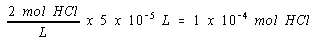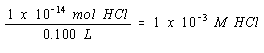Solving Equilibrium Problems Involving Strong Acids

Solving Equilibrium Problems Involving Strong Acids

The simplest acid-base equilibria are those in which a strong acid (or base) is dissolved in water.

As an example, consider the calculation of the pH of a solution formed by adding a single drop of 2 M hydrochloric acid to 100 mL of water.The key to this calculation is remembering that HCl is a strong acid (Ka = 106) and that acids as strong as this can be assumed to dissociate completely in water.

HCl(aq) + H2O(l)H3O+(aq) + Cl-(aq)

The H3O+ ion concentration at equilibrium is therefore essentially equal to the initial concentration of the acid.

A useful rule of thumb says that there are about 20 drops in each milliliter. One drop of 2 M HCl therefore has a volume of 0.05 mL, or 5 x 10-5 L.

The number of moles of HCl added to the water in this calculation can be obtained from the volume and concentration of the hydrochloric acid.The initial concentration of HCl is equal to the number of moles of HCl added to the beaker divided by the volume of water to which the HCl was added.According to this calculation, the initial concentration of HCl is 1 x 10-3 M. If we assume that this acid dissociates completely, the [H3O+] ion concentration at equilibrium is 1 x 10-3 M. The solution prepared by adding one drop of 2 M HCl to 100 mL of water would therefore have a pH of 3.

 pH = - log [H3O+] = - log [1 x 10-3] = -(-3) = 3